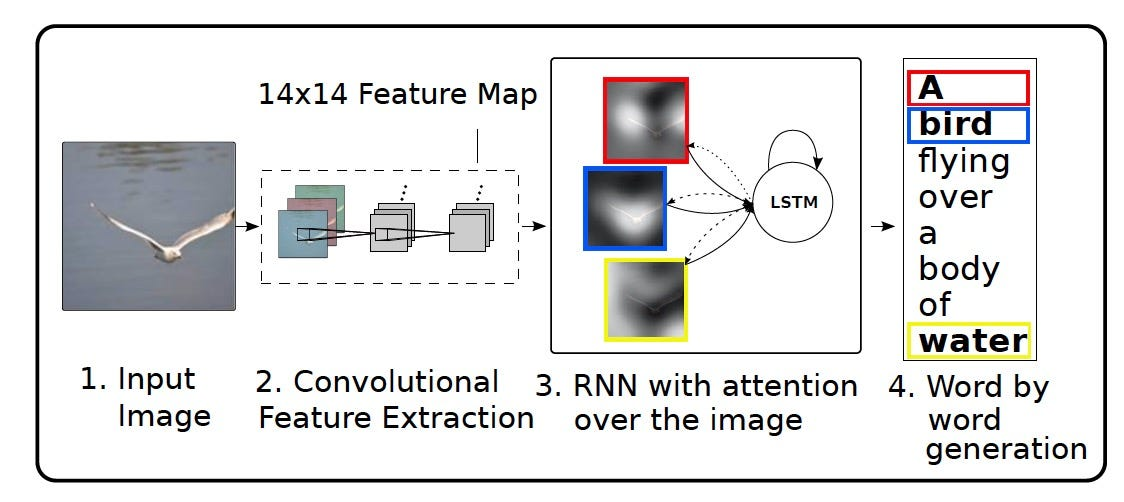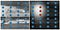# Show, Attend and Tell: Neural Image Caption Generation with Visual Attention

Given an image, the proposed CNN-LSTM network generates image captions. To capture multiple objects inside an image, features are extracted from the lower convolutional layers unlike previous work which use the final fully connected layer. Thus a single image is represented by multiple features a_t at different locations s_t.Approach Overview: In step (2), image features are captured at lower convolutional layers. In step (3), a feature is sampled, fed to LSTM to generate corresponding word. Step 3 is repeated K times to generate K-words caption

The LSTM is trained in a sequence to sequence manner, where a feature a_t, at time t, from location s_t is sampled and fed to LSTM to generates a word. This process is repeated K times to generate K-words image caption.

In this article, I will focus on the Stochastic “Hard” Attention because the soft variant is trivial. I focus on the mathematical formulation of the paper because it is interesting. Thus, the results and computer vision contributions are omitted. In “hard” attention, the location s_t, with feature a_t, is sampled from a multi-nomial distribution defined by parameter alpha. The parameter alpha is learned using a function of the LSTM hidden state h and the image features a — f_att(a,h).

This nice idea is intuitive because at each time step, the LSTM is fed a feature from different image location to generate the corresponding word as shown below

Sampling from a multi-nomial is a trivial process. For example, in the figure below, at time t, location S_1 is more probable than S_3 and S2 and so on.

To imagine how sampling is done at time t, think of image features (a_t) as scattered dot throughout the whole image — blue dots. Given alpha, at time t, some features are more probable to be sampled and fed to the LSTM. For example the red features are more probable than the blue ones in the figure belowLeft: At time t, Features a_t at locations s_t are scattered throughout the image. Right: Given alpha (feature weights) at time t, features, in red, are more probable to be sampled

Yet, sampling within a neural network prevent end-to-end training. Basically the sampling node is random undifferentiable node, so back propagation is infeasible. Reparameterization trick is a typical workaround. Basically, a differentiable surrogate function for sampling is learned. The figure below explains this idea. Instead of random node z, we learn a differentiable function z.

In this paper, the new learnable function is L_s. It is a function of the features a and their locations s , f(s,a), that maximize the probability of the image caption y.

The gradient of this function relative to parameters W in shown the the next figure. Monte Carlo simulation is used to estimate this gradient by substituting with a sampled s_t.

A moving average baseline is used to avoid noisy (jumpy) gradient at each batch.

The soft attention variants is basically a weighted summation of all features a, using alpha as weights. That is why a good understanding for the hard variant will make the soft variant trivial.

I write reviews on computer vision papers. Writing tips are welcomed.

## More from Ahmed Taha

I write reviews on computer vision papers. Writing tips are welcomed.